# Questions and Background¶

Students can either take the Arizona or Goldman-Fristoe test
Question: Can these two tests be combined?
Similar studentss should score the same.

How should we define "similar students"?
For now I will consider the variable included in our population characteristics summary table

Covariate Redcap Variable
Age (Arizona) age_test_aaps
Age (Goldman Firstoe) age_test_gf2
Type of hearing loss type_hl_as, type_hl_ad
Degree of hearing loss degree_hl
Technology tech_as, tech_ad
Gender male
Race race
Primary language spoken prim_lang
Number of children in home sibs
Age of hearing loss diagnosis onset_1
Age first amplified age_amp
Age of intervention age_int
Age enrolled in OPTION age
Length of time in OPTION time
Known cause of hearing loss med_cause=0
Known syndrome synd_cause=0
Another diagnosed disability etiology
Another disability impacts ability to learn etiology_2
Other services outside of OPTION otherserv

How will we asses this?

• Linear model with score as outcome and test type as predictor, controlling for predictors noted above
• If a student took both tests we could asses how well one test score predicts the other

Statistical considerations:

• Many covariates are listed above. Asses sample size to determine degrees of freedom.
• How to handle students with multiple observations?
• For now will take last observation
• How to handle students with both tests?
• For now only looking at students who took one test
• Could try to predict Arizona score form Goldman-Fristoe and vice versa

# Data Prep¶

In :
%matplotlib inline
import matplotlib.pyplot as plt
import pandas as pd
import numpy as np

CHRIS = False

In :
# %pwd

In :
if CHRIS:
print("change path to demographics file")
else:
%run '/Users/alicetoll/Documents/OPTION/LSL-DR/Demographics.ipynb'

False    11601
True      2567
dtype: int64
There are 716 null values for non_english
_mother_ed:
6    5181
4    3022
3    2037
5    1628
2    1421
1     490
0     213
dtype: int64
mother_ed:
1    3458
2    3022
3    1628
0     703
dtype: int64

There are 6073 null values for mother_ed
There are 3494 null values for premature_weeks
0    4627
1       4
2       2
dtype: int64
There are 1715 null values for OAD

hearing_aid:
2    2162
0    1638
1     802
dtype: int64
There are 1768 null values for hearing_aid

cochlear:
0    3081
2     918
1     634
dtype: int64
There are 1715 null values for cochlear
14884
There are 0 null values for tech
_race:
0    7766
2    2528
1    1358
3    1042
6     721
8     527
7     239
4      65
5      33
dtype: int64
race:
0    7766
2    2528
1    1358
4    1346
3    1042
dtype: int64
There are 844 null values for race

/Users/alicetoll/anaconda/envs/py34/lib/python3.3/site-packages/pandas/io/parsers.py:1170: DtypeWarning: Columns (46) have mixed types. Specify dtype option on import or set low_memory=False.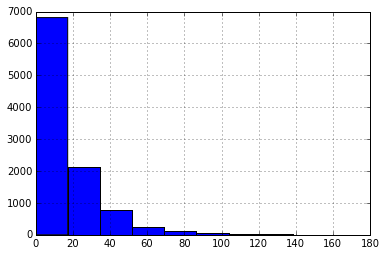In :
if CHRIS:
else:

In :
covar_fields = ['study_id', 'redcap_event_name', 'male', 'race', 'prim_lang', 'sib', 'onset_1',
'age_amp', 'age_int', 'age', 'time', 'med_cause', 'synd_cause', 'etiology', 'etiology_2',

covar = demographic[covar_fields]

# Need to create vars and add:
# - type of hearing loss
# - degree of hearing loss
# - technology
# - CI Manufacturer

# Do we want to collapse race?

# Age is in articulation_fields
# - age_test_aaps = Arizona
# - age_test_gf2 = Goldman-Fristoe

In :
# Type of hearing loss
# - Bilateral SNHL
# - Unilateral SNHL
# - Bilateral Auditory Neuropathy
# - Unilateral Auditory Neuropathy

covar['bilateral_snhl'] = 0
covar.loc[(covar.type_hl_ad == 0) & (covar.type_hl_as == 0), 'bilateral_snhl'] = 1

covar['unilateral_snhl'] = 0
covar.loc[(covar.type_hl_ad == 0) & (covar.type_hl_as == 4), 'unilateral_snhl'] = 1
covar.loc[(covar.type_hl_ad == 4) & (covar.type_hl_as == 0), 'unilateral_snhl'] = 1

covar['bilateral_an'] = 0
covar.loc[(covar.type_hl_ad == 3) & (covar.type_hl_as == 3), 'bilateral_an'] = 1

covar['unilateral_an'] = 0
covar.loc[(covar.type_hl_ad == 0) & (covar.type_hl_as == 3), 'unilateral_an'] = 1
covar.loc[(covar.type_hl_ad == 3) & (covar.type_hl_as == 0), 'unilateral_an'] = 1

/Users/alicetoll/anaconda/envs/py34/lib/python3.3/site-packages/IPython/kernel/__main__.py:7: SettingWithCopyWarning:
A value is trying to be set on a copy of a slice from a DataFrame.
Try using .loc[row_indexer,col_indexer] = value instead

See the the caveats in the documentation: http://pandas.pydata.org/pandas-docs/stable/indexing.html#indexing-view-versus-copy
/Users/alicetoll/anaconda/envs/py34/lib/python3.3/site-packages/pandas/core/indexing.py:415: SettingWithCopyWarning:
A value is trying to be set on a copy of a slice from a DataFrame.
Try using .loc[row_indexer,col_indexer] = value instead

See the the caveats in the documentation: http://pandas.pydata.org/pandas-docs/stable/indexing.html#indexing-view-versus-copy
self.obj[item] = s
/Users/alicetoll/anaconda/envs/py34/lib/python3.3/site-packages/IPython/kernel/__main__.py:10: SettingWithCopyWarning:
A value is trying to be set on a copy of a slice from a DataFrame.
Try using .loc[row_indexer,col_indexer] = value instead

See the the caveats in the documentation: http://pandas.pydata.org/pandas-docs/stable/indexing.html#indexing-view-versus-copy
/Users/alicetoll/anaconda/envs/py34/lib/python3.3/site-packages/IPython/kernel/__main__.py:14: SettingWithCopyWarning:
A value is trying to be set on a copy of a slice from a DataFrame.
Try using .loc[row_indexer,col_indexer] = value instead

See the the caveats in the documentation: http://pandas.pydata.org/pandas-docs/stable/indexing.html#indexing-view-versus-copy
/Users/alicetoll/anaconda/envs/py34/lib/python3.3/site-packages/IPython/kernel/__main__.py:17: SettingWithCopyWarning:
A value is trying to be set on a copy of a slice from a DataFrame.
Try using .loc[row_indexer,col_indexer] = value instead

See the the caveats in the documentation: http://pandas.pydata.org/pandas-docs/stable/indexing.html#indexing-view-versus-copy

In :
# - Bilateral SNHL
# - Unilateral SNHL
# - Bilateral Auditory Neuropathy
# - Unilateral Auditory Neuropathy

In :
bi_snhl = covar.bilateral_snhl == 1
uni_snhl = covar.unilateral_snhl == 1
bi_an = covar.bilateral_an == 1
uni_an = covar.unilateral_an == 1

covar.loc[bi_snhl, 'type_hl'] = "bi_snhl"
covar.loc[uni_snhl, 'type_hl'] = "uni_snhl"
covar.loc[bi_an, 'type_hl'] = "bi_an"
covar.loc[uni_an, 'type_hl'] = "uni_an"

/Users/alicetoll/anaconda/envs/py34/lib/python3.3/site-packages/pandas/core/indexing.py:249: SettingWithCopyWarning:
A value is trying to be set on a copy of a slice from a DataFrame.
Try using .loc[row_indexer,col_indexer] = value instead

See the the caveats in the documentation: http://pandas.pydata.org/pandas-docs/stable/indexing.html#indexing-view-versus-copy
self.obj[key] = _infer_fill_value(value)

In :
articulation_fields = ['study_id','redcap_event_name', 'age_test_aaps','aaps_ss','age_test_gf2','gf2_ss']
articulation = lsl_dr_project.export_records(fields=articulation_fields, format='df',
df_kwargs={'index_col':None,
'na_values':[999, 9999]})

In :
# articulation.head()

In :
# create indicator variable if a student took either test
articulation.loc[articulation.aaps_ss.notnull() | articulation.gf2_ss.notnull(), 'test'] = 1
# drop observations when neither test was taken
temp = articulation.dropna(subset = ['test'])
# Can drop test variable
temp = temp.drop('test', 1)
# Create new variable if student took both tests in one observation
temp['both'] = 0
temp.loc[temp.aaps_ss.notnull() & temp.gf2_ss.notnull(), 'both'] = 1
print(temp.both.value_counts()) # 73 students took both tests, 5716 took only one

0    5760
1      73
dtype: int64


Merge covariates and articulation data frames

In :
# merge
temp2 = pd.merge(temp, covar, on=['study_id', 'redcap_event_name'])

In :
AAPS = temp2.aaps_ss.notnull()
GF2 = temp2.gf2_ss.notnull()

temp2.loc[AAPS, "test_name"] = "AAPS"
temp2.loc[GF2, "test_name"] = "GF2"


Create datasets which contain students who either took one or both tests in a single year. If a student has multiple observations, take the last observation in each dataset.

In :
# One test
single = temp2.loc[temp2.both==0,]
a = single.shape
single = single.groupby('study_id').last()
b = single.shape
print('We have', a, 'observations where a student took one test in a single year, but only', b, 'unique students')

# Both tests
both = temp2.loc[temp2.both==1,]
a = both.shape
both = both.groupby('study_id').last()
b = both.shape
print('We have', a, 'observations where a student took both test in a single year, but only', b, 'unique students')

We have 5760 observations where a student took one test in a single year, but only 2730 unique students
We have 73 observations where a student took both test in a single year, but only 55 unique students

In :
del temp
del temp2


# Students who only took one test (within a single year)¶

In :
# Create variables to hold test score and age, and indicator for test name

AAPS = single.aaps_ss.notnull()
GF2 = single.gf2_ss.notnull()

single.loc[AAPS, "test_name"] = "AAPS"
single.loc[GF2, "test_name"] = "GF2"

single.loc[AAPS, "score"] = single.aaps_ss
single.loc[GF2, "score"] = single.gf2_ss

single.loc[AAPS, "age_test"] = single.age_test_aaps
single.loc[GF2, "age_test"] = single.age_test_gf2

# single = single.drop(['age_test_aaps', 'aaps_ss', 'age_test_gf2', 'gf2_ss', 'both'], 1)

In :
# Number of observations
single.shape

Out:
2730
In :
# Missingness
len(single.index)-single.count()

Out:
redcap_event_name       0
age_test_aaps        2448
aaps_ss              2448
age_test_gf2          181
gf2_ss                180
both                    0
male                   22
race                   69
prim_lang              42
sib                   247
onset_1               913
age_amp               973
age_int              1063
age                    46
time                  170
med_cause              57
synd_cause             60
etiology               48
etiology_2            527
otherserv             974
type_hl_as            409
bilateral_snhl          0
unilateral_snhl         0
bilateral_an            0
unilateral_an           0
type_hl               780
test_name               0
score                   0
age_test                1
dtype: int64

## Plots¶

In :
%matplotlib inline
import seaborn as sns
# sns.set(style="white", color_codes=True)

In :
width = 3
sns.kdeplot(np.array(single[single.test_name == 'GF2'].score), bw=width, label = "Goldman-Fristoe")
sns.kdeplot(np.array(single[single.test_name == 'AAPS'].score), bw=width, label = "Arizona")

Out:
<matplotlib.axes._subplots.AxesSubplot at 0x10dcc5850>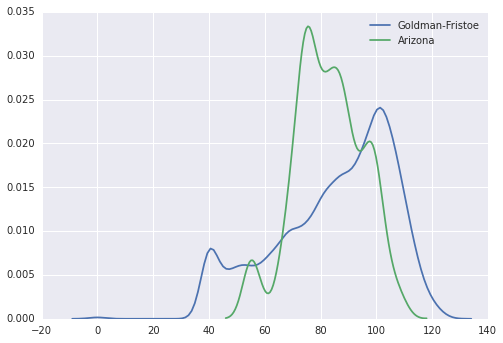In :
single.type_hl.value_counts()

Out:
bi_snhl     1811
bi_an         73
uni_snhl      57
uni_an         9
dtype: int64

We may want to consider looking at just bilateral SNHL vs other based on sample size.

In :
# Kernal Density Estimates broken out by type of hearing loss

plt.figure()

# Bilateral SNHL
plt.subplot(2,2,1)
sns.kdeplot(np.array(single.loc[(single.test_name == 'GF2') & (single.bilateral_snhl == 1), 'score']),
bw=width)
sns.kdeplot(np.array(single.loc[(single.test_name == 'AAPS') & (single.bilateral_snhl == 1), 'score']),
bw=width)
plt.title('Bilateral SNHL')
plt.ylabel('Density')
plt.xlabel('Standardized Score')

# Unilateral SNHL
plt.subplot(2,2,2)
sns.kdeplot(np.array(single.loc[(single.test_name == 'GF2') & (single.unilateral_snhl == 1), 'score']),
bw=width)
sns.kdeplot(np.array(single.loc[(single.test_name == 'AAPS') & (single.unilateral_snhl == 1), 'score']),
bw=width)
plt.title('Unilateral SNHL')
plt.ylabel('Density')
plt.xlabel('Standardized Score')

# Bilateral AN
plt.subplot(2,2,3)
sns.kdeplot(np.array(single.loc[(single.test_name == 'GF2') & (single.bilateral_an == 1), 'score']),
bw=width, label = "Goldman-Fristoe")
sns.kdeplot(np.array(single.loc[(single.test_name == 'AAPS') & (single.bilateral_an == 1), 'score']),
bw=width, label = "Arizona")
plt.title('Bilateral Auditory Neuropathy')
plt.ylabel('Density')
plt.xlabel('Standardized Score')

# Unilateral AN
plt.subplot(2,2,4)
sns.kdeplot(np.array(single.loc[(single.test_name == 'GF2') & (single.unilateral_an == 1), 'score']),
bw=width)
# no obs for Arizona with Unilateral AN
# sns.kdeplot(np.array(single.loc[(single.test_name == 'AAPS') & (single.unilateral_an == 1), 'score']),
#                bw=width, label = "Arizona")
plt.title('Unilateral Auditory Neuropathy')
plt.ylabel('Density')
plt.xlabel('Standardized Score')

plt.tight_layout()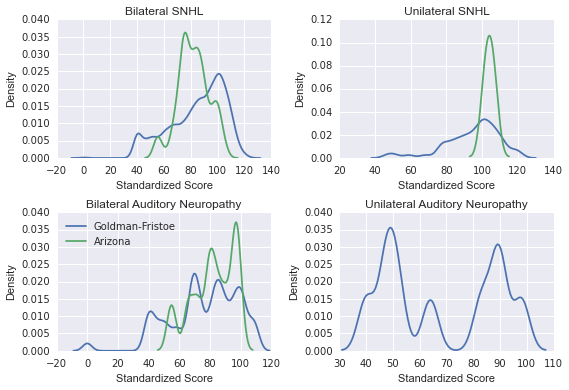### Want to make this function¶

def mykdeplot(df, var, width): sns.kdeplot(np.array(getattr(df[df.Group == 'a'], var)), bw=width, label = "Group A") sns.kdeplot(np.array(getattr(df[df.Group == 'b'], var)), bw=width, label = "Group B")def mykdeplot(df, var, width): sns.kdeplot(np.array(getattr(df[(artShort1.test_name == 'GF2') & (df.var == 1)], 'score')), bw=width, label = "Goldman-Fristoe") sns.kdeplot(np.array(getattr(artShort1[(df.test_name == 'AAPS') & (df.var == 1)], 'score')), bw=width, label = "Arizona")mykdeplot(single, 'bilateral_an', 5)

## Linear Model¶

# https://github.com/justmarkham/DAT4/blob/master/notebooks/08_linear_regression.ipynb # this is the standard import if you're using "formula notation" (similar to R) import statsmodels.formula.api as smf # create a fitted model in one line lm = smf.ols(formula='score ~ test_name + age_test + male', data=artShort1).fit() # print the coefficients print(lm.params) # print the confidence intervals for the model coefficients print(lm.conf_int())
In :
import statsmodels.formula.api as smf


Linear model predicting score from test name, age, gender, and type of hearing loss.

In :
lm = smf.ols(formula="""score ~ test_name + age_test + male +
bilateral_snhl + unilateral_snhl + bilateral_an + unilateral_an""",
data=single).fit()
lm.summary()

Out:
Dep. Variable: R-squared: score 0.019 OLS 0.016 Least Squares 7.470 Sun, 17 Apr 2016 6.29e-09 18:46:52 -12016. 2707 2.405e+04 2699 2.409e+04 7 nonrobust
coef std err t P>|t| [95.0% Conf. Int.] 87.8178 1.979 44.369 0.000 83.937 91.699 1.4962 1.589 0.942 0.346 -1.619 4.612 -0.0570 0.013 -4.545 0.000 -0.082 -0.032 0.1904 0.792 0.241 0.810 -1.362 1.742 -0.5525 0.880 -0.628 0.530 -2.279 1.174 9.0884 2.868 3.169 0.002 3.465 14.711 -7.9264 2.547 -3.112 0.002 -12.921 -2.932 -17.2806 6.879 -2.512 0.012 -30.769 -3.792
 Omnibus: Durbin-Watson: 180.329 1.756 0 204.964 -0.653 3.11e-45 2.666 1380

From the output above, we see test_name is not significant (p = 0.334). This tells us that after accounting for the student's age when taking the test, gender, and type of hearing loss, knowing which test the student took does not help us to predict there score. This suggests that similar students score the same regardless of which test they took.

We need to determine which variables should be included in our model.

# Students who only took both tests (within a single year)¶

## Introduction¶

We currently treat standardized Arizona and Goldman-Fristoe tests scores the same. The goal of this analysis is to relate the two tests so they can be transformed for future modeling purposes.

We only have 55 observations (before accounting for missingness). That only leaves us with 3-6 degress of freedom for modeling. A continuous variable will use up one degree of freedom, and the number of deggrees of freedom used by a categorical variable is one less than the number of categories.

My initial intuition was to model Arizona score as a function of Goldman-Fristoe score, age, type of hearing loss, and whether or not the student receives other learning servies. Unfortunatly on this small sample size the observations for type of hearing loss and other serivces are rather homongenous, so I do not believe they will be very helpful in predicted Arizona score.

We may want to allow for nonlinearity by including a spline term for the Goldman-Fristoe score. A spline with 3 knots would use 3 degrees of freedom.

The candidate models considered are sumarized in the subsection titled Models.

Variables that were eliminated from consideration due to homogenity

• type_hl (47 out of 55 students have bilateral SNHL)
• etiology (42 out of 55 have another diagnosed disability)
• otherserv (35 out of 55 do not recieve other educational services, 12 out of 55 are missing)

Response: Arizona scores (aaps_ss)

Considered Covariate Redcap Variable Degrees of Freedom
Goldman Fristoe Score gf2_ss 1
Goldman Fristoe Score, spline gf2_ss 3
Age (Arizona) age_test_aaps 1
Age (Goldman Firstoe) age_test_gf2 1
Gender male 1
Race (White/Non-White) white 1
Age of hearing loss diagnosis onset_1 1
Length of time in OPTION time 1
Another disability impacts ability to learn etiology_impact 1

## Potential variables for modelings¶

In :
# With 55 observations (before missingness), we really only have 3-6 degrees of freedom to use in a model.
both.shape

Out:
(55, 28)
In :
# Arizona is more conservative towards "normal" (85)
# May want to consider a spline for Goldman-Fristoe
width = 4
sns.kdeplot(np.array(both.gf2_ss), bw=width, label = "Goldman-Fristoe")
sns.kdeplot(np.array(both.aaps_ss), bw=width, label = "Arizona")
plt.ylabel('Density')
plt.xlabel('Standardized Score')

Out:
<matplotlib.text.Text at 0x10c25ae10>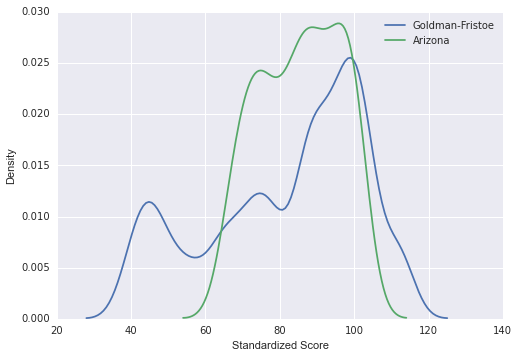In :
# Large age range (in months).  May want to use a spline for age
# Don't have enough degrees of freedom to spline both variables
sns.kdeplot(both.age_test_gf2, bw=5, label = "Goldman-Fristoe")
sns.kdeplot(both.age_test_aaps, bw=5, label = "Arizona")
plt.ylabel('Density')
plt.xlabel('Age (months)')

Out:
<matplotlib.text.Text at 0x1110c81d0>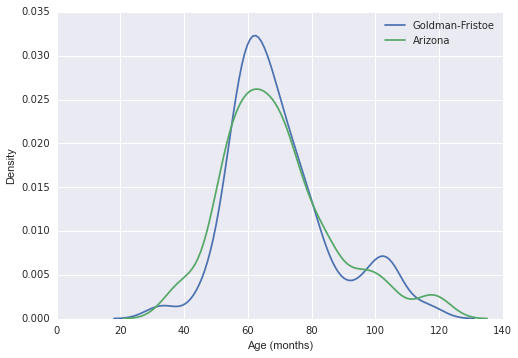In :
# Race has a lot of categories
# To preserve degrees of freedom we can reduce to white/non-white
both.race.value_counts(dropna=False, sort=False)
# 0 = Cacucasian
# 1 = Black or African American
# 2 = Hispanic or Latino
# 3 = Asian
# 4 = Native Hawaiians/Pacific Islanders
# 5 = American Indian/Alaska Natives
# 6 = Multiracial
# 7 = Unknown
# 8 = Other

Out:
NaN     2
0     26
1      6
2      8
3      4
4      9
dtype: int64
In :
both['white'] = np.nan
both.loc[(both.race == 0), 'white'] = 1
both.loc[(both.race !=0) & (pd.notnull(both.race)), 'white'] = 0
both.white.value_counts(dropna=False, sort=False)

Out:
NaN     2
0     27
1     26
dtype: int64
In :
# Etiology 2: impact of the other condition(s) that contribute to the child's overall learning abilities
# To preserve degrees of freedom we can reduce to impacts (1, 2, 3) and doesn't imact (0, 4)
both.etiology_2.value_counts(dropna=False, sort=False)
# 0 = None
# 1 = Mild
# 2 = Moderate
# 3 = Severe
# 4 = N/A (no additional disability suspected or identified)

Out:
NaN     1
0     11
1      5
2     12
4     26
dtype: int64
In :
both['etiology_impact'] = np.nan
both.loc[(both.etiology_2 == 0) | (both.etiology_2 == 4), 'etiology_impact'] = 0
both.loc[(both.etiology_2 == 1) | (both.etiology_2 == 2) | (both.etiology_2 == 3), 'etiology_impact'] = 1
both.etiology_impact.value_counts(dropna=False)

Out:
 0     37
1     17
NaN     1
dtype: int64
In :
# Amount of time enrolled in OPTION: 2 missing obs
len(both.time.index)-both.time.count()

Out:
2
In :
# Age of onset: 10 missing obs
# This is a lot of observations to sacrifice
# Would be good if we could use multiple imputation
len(both.onset_1.index)-both.onset_1.count()

Out:
10

## Variables eliminated due to heterogeneity¶

In :
# Most of the students who took both tests have bilateral SNHL
# 4 observations are missing
# Because a large majority of the students only have bilateral SNHL, it will not be a useful predictor.
both.type_hl.value_counts(dropna=False)

Out:
bi_snhl     47
NaN          4
bi_an        3
uni_snhl     1
dtype: int64
In :
# 42 out of 55 do not have another diagnosed disability
# note: this doesn't agree with etiology_2 coding
both.etiology.value_counts(dropna=False, sort=False)

Out:
0     9
1    42
2     1
3     3
dtype: int64
In :
# Other services: 12 missing obs
# Most students do not recieve other services
both.otherserv.value_counts(dropna=False, sort=False)
# 0 = Yes
# 1 = No
# 2 = Unknown

Out:
NaN    12
0      3
1     35
2      5
dtype: int64

## Models¶

Model Name Variables n obs df R-squared
m1 gf2_ss + age_test_gf2 + age_test_aaps + male + white + etiology_impact 52 6 0.782
m2 gf2_ss + age_test_gf2 + age_test_aaps 55 3 0.771
m3 bs(gf2_ss, 3) + age_test_gf2 + age_test_aaps 55 5 0.781
m4 cr(gf2_ss, df=3, constraints='center') + age_test_gf2 + age_test_aaps 55 5 0.780
m5 gf2_ss + age_test_gf2 + age_test_aaps + time + onset_1 45 5 0.877
m6 gf2_ss + age_test_gf2 + age_test_aaps + time 52 4 0.819
In :
m1 = smf.ols(formula="""aaps_ss ~ gf2_ss + age_test_gf2 + age_test_aaps +
male + white + etiology_impact""",
data=both).fit()
m1.summary()

Out:
Dep. Variable: R-squared: aaps_ss 0.782 OLS 0.753 Least Squares 26.85 Sun, 17 Apr 2016 2.44e-13 18:46:54 -158.30 52 330.6 45 344.3 6 nonrobust
coef std err t P>|t| [95.0% Conf. Int.] 53.1924 5.154 10.320 0.000 42.811 63.574 0.4439 0.038 11.787 0.000 0.368 0.520 -0.3120 0.147 -2.125 0.039 -0.608 -0.016 0.2512 0.134 1.881 0.066 -0.018 0.520 0.5687 1.645 0.346 0.731 -2.745 3.882 1.7043 1.638 1.041 0.304 -1.594 5.003 -2.0195 1.702 -1.187 0.242 -5.447 1.408
 Omnibus: Durbin-Watson: 2.316 2.265 0.314 1.555 -0.403 0.46 3.263 880
In :
m2 = smf.ols(formula="""aaps_ss ~ gf2_ss + age_test_gf2 + age_test_aaps""",
data=both).fit()
m2.summary()

Out:
Dep. Variable: R-squared: aaps_ss 0.771 OLS 0.757 Least Squares 57.12 Sun, 17 Apr 2016 2.52e-16 18:46:54 -168.24 55 344.5 51 352.5 3 nonrobust
coef std err t P>|t| [95.0% Conf. Int.] 54.8203 4.437 12.354 0.000 45.912 63.729 0.4339 0.034 12.623 0.000 0.365 0.503 -0.3497 0.135 -2.588 0.013 -0.621 -0.078 0.2834 0.124 2.280 0.027 0.034 0.533
 Omnibus: Durbin-Watson: 2.865 2.272 0.239 2.027 -0.442 0.363 3.32 798
In :
# Use to print anova tables (for when we use splines)
import statsmodels.api as sm

In :
# bs() B-spline
m3 = smf.ols(formula="""aaps_ss ~ bs(gf2_ss, 3) + age_test_gf2 + age_test_aaps""",
data=both).fit()
m3.summary()

Out:
Dep. Variable: R-squared: aaps_ss 0.781 OLS 0.759 Least Squares 35.01 Sun, 17 Apr 2016 4.62e-15 18:46:54 -166.93 55 345.9 49 357.9 5 nonrobust
coef std err t P>|t| [95.0% Conf. Int.] 75.4249 4.181 18.040 0.000 67.023 83.827 -1.3268 7.914 -0.168 0.868 -17.230 14.576 22.9797 5.155 4.458 0.000 12.620 33.339 27.7088 4.170 6.645 0.000 19.330 36.088 -0.3629 0.136 -2.671 0.010 -0.636 -0.090 0.2951 0.126 2.342 0.023 0.042 0.548
 Omnibus: Durbin-Watson: 3.445 2.254 0.179 2.473 -0.464 0.29 3.465 1300
In :
sm.stats.anova_lm(m3, typ=2)

Out:
sum_sq df F PR(>F)
bs(gf2_ss, 3) 4634.360674 3 54.312079 1.308633e-15
age_test_gf2 202.877916 1 7.132842 1.024204e-02
age_test_aaps 156.014750 1 5.485213 2.328615e-02
Residual 1393.696551 49 NaN NaN
In :
# cr() natural cubic spline
m4 = smf.ols(formula="""aaps_ss ~ cr(gf2_ss, df=3, constraints='center') + age_test_gf2 + age_test_aaps""",
data=both).fit()
m4.summary()

Out:
Dep. Variable: R-squared: aaps_ss 0.780 OLS 0.757 Least Squares 34.68 Sun, 17 Apr 2016 5.52e-15 18:46:54 -167.14 55 346.3 49 358.3 5 nonrobust
coef std err t P>|t| [95.0% Conf. Int.] 90.0725 3.236 27.838 0.000 83.570 96.575 -0.6435 1.915 -0.336 0.738 -4.491 3.204 14.6513 1.804 8.121 0.000 11.026 18.277 16.6059 2.660 6.243 0.000 11.261 21.951 -0.3580 0.137 -2.621 0.012 -0.633 -0.083 0.2940 0.127 2.321 0.025 0.039 0.549
 Omnibus: Durbin-Watson: 2.991 2.239 0.224 2.083 -0.432 0.353 3.404 460
In :
sm.stats.anova_lm(m4, typ=2)

Out:
sum_sq df F PR(>F)
cr(gf2_ss, df=3, constraints='center') 4624.005992 3 53.791079 1.567175e-15
age_test_gf2 196.774953 1 6.867251 1.165756e-02
age_test_aaps 154.307597 1 5.385183 2.451412e-02
Residual 1404.051233 49 NaN NaN
In :
m5 = smf.ols(formula="""aaps_ss ~ gf2_ss + age_test_gf2 + age_test_aaps +
time + onset_1""",
data=both).fit()
m5.summary()

Out:
Dep. Variable: R-squared: aaps_ss 0.877 OLS 0.862 Least Squares 55.82 Sun, 17 Apr 2016 9.93e-17 18:46:54 -122.00 45 256.0 39 266.8 5 nonrobust
coef std err t P>|t| [95.0% Conf. Int.] 63.0834 3.795 16.622 0.000 55.407 70.760 0.3897 0.029 13.285 0.000 0.330 0.449 -0.5494 0.113 -4.871 0.000 -0.778 -0.321 0.3424 0.103 3.327 0.002 0.134 0.551 1.8769 0.523 3.590 0.001 0.819 2.934 0.0459 0.049 0.941 0.353 -0.053 0.144
 Omnibus: Durbin-Watson: 0.832 2.356 0.66 0.209 -0.008 0.901 3.333 847
In :
m6 = smf.ols(formula="""aaps_ss ~ gf2_ss + age_test_gf2 + age_test_aaps + time""",
data=both).fit()
m6.summary()

Out:
Dep. Variable: R-squared: aaps_ss 0.819 OLS 0.804 Least Squares 54.32 Sun, 17 Apr 2016 3.13e-17 18:46:54 -155.96 53 321.9 48 331.8 4 nonrobust
coef std err t P>|t| [95.0% Conf. Int.] 58.6568 4.169 14.069 0.000 50.274 67.040 0.3976 0.033 12.071 0.000 0.331 0.464 -0.4447 0.127 -3.510 0.001 -0.699 -0.190 0.3094 0.114 2.719 0.009 0.081 0.538 1.5959 0.539 2.963 0.005 0.513 2.679
 Omnibus: Durbin-Watson: 5.319 2.411 0.07 4.242 -0.632 0.12 3.567 819

## Plots¶

In :
import scipy
# (correlation, p-value)
pc = scipy.stats.pearsonr(both.gf2_ss, both.aaps_ss)
print("Peasron Corelation: " "%.3f" % pc)
sc = scipy.stats.spearmanr(both.gf2_ss, both.aaps_ss)
print("Spearman Corelation: " "%.3f" % sc)

Peasron Corelation: 0.860
Spearman Corelation: 0.848

In :
sns.lmplot("gf2_ss", "aaps_ss", data=both, fit_reg=False)
sns.regplot(both.gf2_ss, both.aaps_ss, scatter=False, lowess=True, label='LOWESS')
plt.plot([50, 110], [50, 110], 'red', label='Identity');
legend = plt.legend(loc='upper left')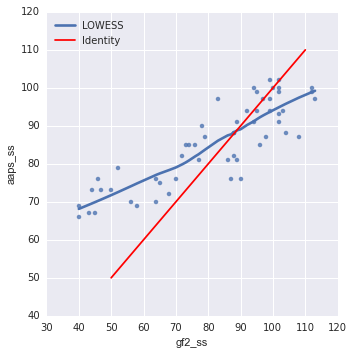In :
# pearson correlation
g = sns.jointplot(x="gf2_ss", y="aaps_ss", data=both, marginal_kws=dict(bins=15, rug=True))
# for spearman correlation, add 'stat_func=spearmanr' to joinplot()
x0, x1 = g.ax_joint.get_xlim()
y0, y1 = g.ax_joint.get_ylim()
lims = [max(x0, y0)-10, min(x1, y1)+10]
g.ax_joint.plot(lims, lims, ':k')

Out:
[<matplotlib.lines.Line2D at 0x10f7d9f10>]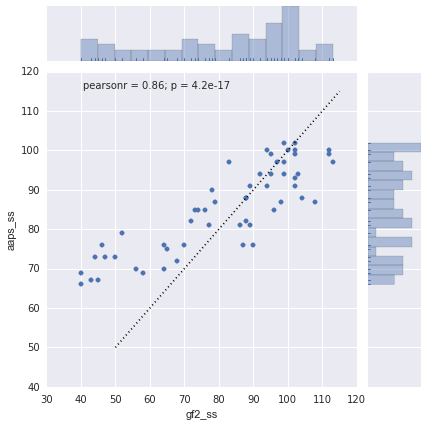In :
# regression line with confidence bands
sns.jointplot(x="gf2_ss", y="aaps_ss", data=both, kind="reg", marginal_kws=dict(bins=15, rug=True));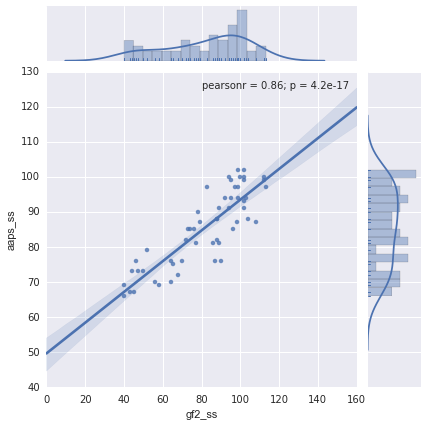In :
fig = plt.figure(figsize=(12,15))
fig = sm.graphics.plot_regress_exog(m1, 'gf2_ss', fig=fig)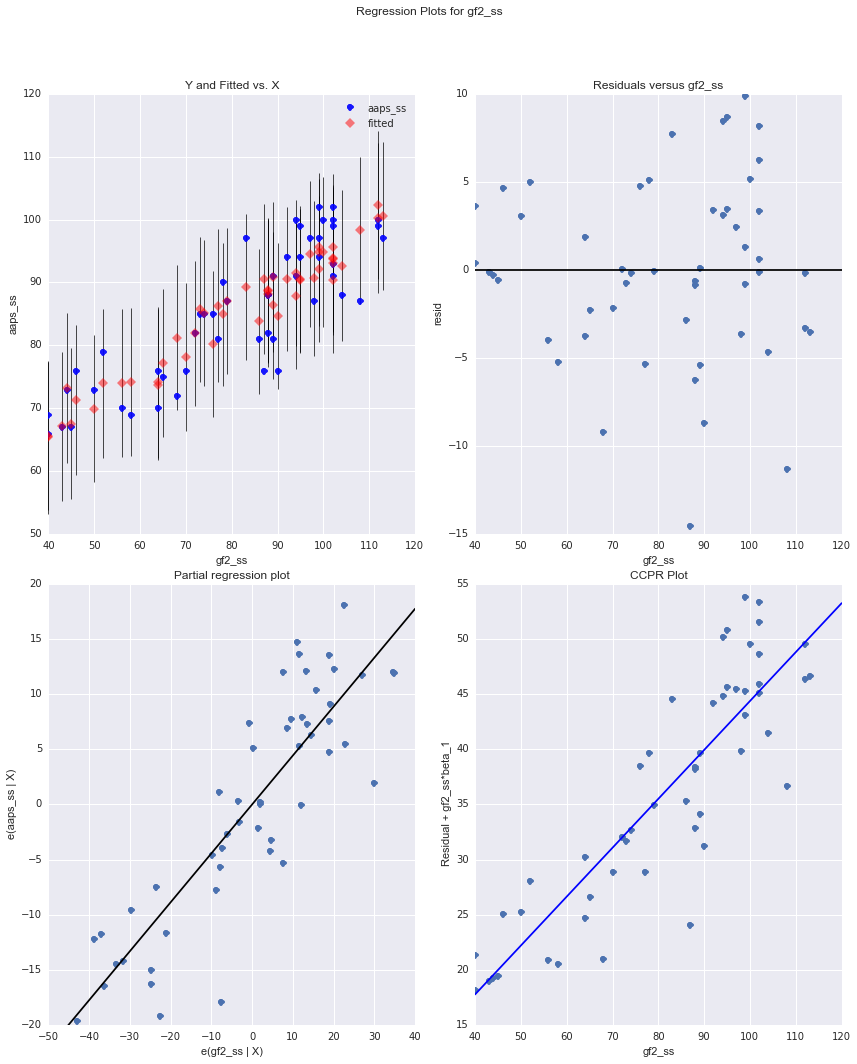In :
fig, ax = plt.subplots(figsize=(8,6))
ax.plot(np.array(both.aaps_ss), m3.fittedvalues, 'r.')
plt.plot([65, 105], [65, 105], 'blue', label='Identity')
legend = plt.legend(loc='upper left')
plt.xlabel('Observed Arizona Score')
plt.ylabel('Predicted Arizona Score')
plt.title('Model 3')

Out:
<matplotlib.text.Text at 0x10ec3e6d0>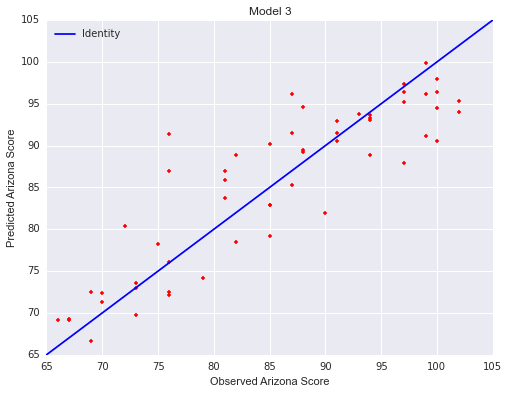In :
fig, ax = plt.subplots(figsize=(8,6))
ax.plot(np.array(both.gf2_ss), m3.fittedvalues, 'r.', label='Predicted')
ax.plot(np.array(both.gf2_ss), np.array(both.aaps_ss), 'g.', label='Observed')
plt.plot([35, 110], [35, 110], 'blue', label='Identity');
legend = plt.legend(loc='upper left')
plt.xlabel('Goldman-Fristoe Score')
plt.ylabel('Arizona Score')
plt.title('Model 3')
ax.set_xlim([30,120])
ax.set_ylim([60,110])

Out:
(60, 110)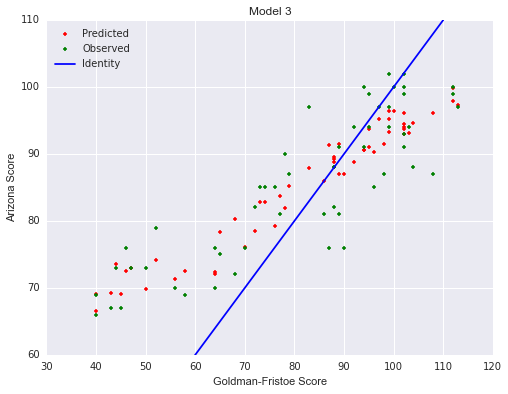In :
aaps_m6 = both[both['time'].notnull()].aaps_ss
gf2_m6 = both[both['time'].notnull()].gf2_ss

In :
fig, ax = plt.subplots(figsize=(8,6))
ax.plot(aaps_m6, m6.fittedvalues, 'r.')
plt.plot([65, 105], [65, 105], 'blue', label='Identity')
legend = plt.legend(loc='upper left')
plt.xlabel('Observed Arizona Score')
plt.ylabel('Predicted Arizona Score')
plt.title('Model 6')

Out:
<matplotlib.text.Text at 0x10f10d190>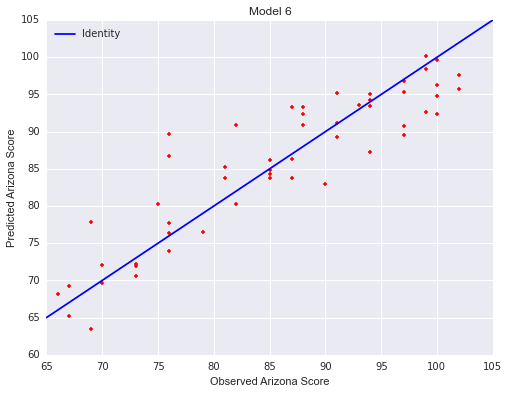In :
fig, ax = plt.subplots(figsize=(8,6))
ax.plot(gf2_m6, m6.fittedvalues, 'r.', label='Predicted')
ax.plot(gf2_m6, aaps_m6, 'g.', label='Observed')
plt.plot([35, 110], [35, 110], 'blue', label='Identity');
legend = plt.legend(loc='upper left')
plt.xlabel('Goldman-Fristoe Score')
plt.ylabel('Arizona Score')
plt.title('Model 6')
ax.set_xlim([30,120])
ax.set_ylim([60,110])

Out:
(60, 110)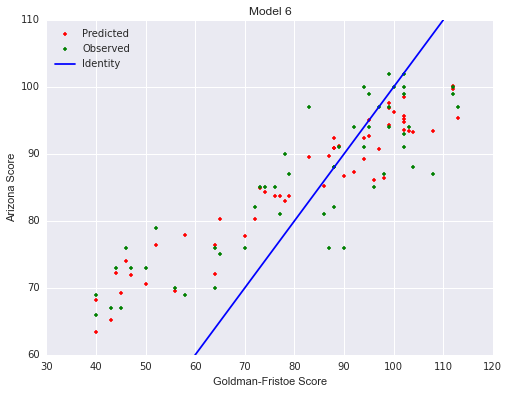## Future Considerations¶

In an effort to increase sample size, we could model all observations with both scores (73) and use clustered robust standard errors or build a repeated measures model.

For robust standard errors see http://statsmodels.sourceforge.net/0.6.0/stats.html
sandwich_covariance.cov_cluster(results, group)